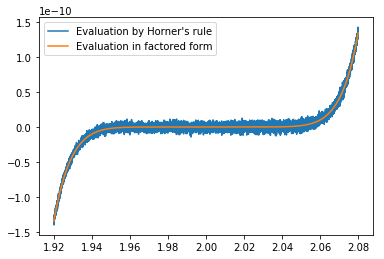Floating Point Error in Horner's Rule Polynomial Evaluation

The following example is taken from Applied Numerical Linear Algebra by James Demmel, SIAM 1997.

Here are three methods for evaluating the function

$$f(x)=(x-2)^9 = -512 + 2304x -4608x^2 +5476x^3 -4032x^4 +2016x^5-672x^6+144x^7-18x^8+x^9$$
In :
import numpy as np

#Evalue  polynomial in factored form
def f(x):
return (x-2.)**9

#coefficients for expanded form
coeffs = np.asarray([-512., 2304., -4608., 5376., -4032., 2016., -672., 144., -18., 1.])

#Evaluate polynomial using coefficients
def p(x):
return np.inner(coeffs, np.asarray([x**i for i in range(10)]))

#Evaluate Horner's rule for polynomial
def h(x):
y = 0.
#[::-1] looks at all elements with stride -1, reversing the order
for c in coeffs[::-1]:
y = x*y+c
return y

#Define 8000 points between 1.92 and 2.08
xpts = 1.92+np.arange(8000.)/50000.

import matplotlib
import matplotlib.pyplot as pt

#plot functions evaluated at each point using Horder's rule and using factored form
pt.plot(xpts,[h(x) for x in xpts],label='Evaluation by Horner\'s rule')
pt.plot(xpts,[f(x) for x in xpts],label='Evaluation in factored form')
pt.legend()
pt.show()It seems Horner's rule is inaccurate when $f(x)\approx 0$, lets try to understand why.

The first method uses $(x-2)^9$ directly, encurring a backward error of $\epsilon$ (machine epsilon), since given $z=\textit{fl}(x+2)$, we can obtain $z^9$ to the same precision, therby solving the problem for $\hat{x}=\textit{fl}(x+2)-2=x+ \Delta x$, $|\Delta x|\leq \epsilon$.

The second uses the inner product formula $$f(x)=p(x)= \sum_{i=0}^9c_ix^i =\begin{bmatrix} -512 & 2304 & -4608 & 5476 & -4032 & 2016 & -672 & 144 & 18& 1 \end{bmatrix}\begin{bmatrix} 1\\ x \\ x^2 \\ x^3 \\ x^4 \\ x^5 \\ x^6 \\ x^7 \\ x^8 \\ x^9 \end{bmatrix}$$

The third uses Horner's rule, which requires fewer operations

$$f(x)=h(x) = c_0 + (c_1 + \ldots (c_8 + c_9x)x \ldots )x$$

Each addition in the last two methods incurs a relative error of at most $\epsilon$. An error in the innermost parenthesis, would correspond to evaluating the function at a slightly perturbed $x$. However, the error in the summation done last, contributes directly to the result. When $\left|f(x)\right|<|c_0|\epsilon$, the result will contain no accurate significant digits.

In terms of backward stability, extrapolating the above argument implies that the backward absolute error bound has the bound $$\textit{fl}(h(x))-f(x)=\Delta x \leq \epsilon \left(1+\left|\frac{df^{-1}}{dx}(x)\right|\right).$$

More generally, given their factorized form, we can evaluate any function with unconditional backward stability. But factorization is hard!

In [ ]: